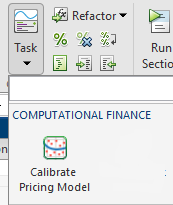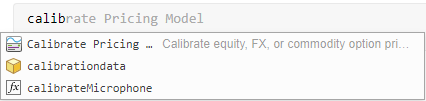# Calibrate Pricing Model

Calibrate option pricing model in the Live Editor

Since R2022a

## Description

The Calibrate Pricing Model task lets you interactively calibrate an equity, FX, or commodity option pricing model using market data. The task automatically generates MATLAB® code for your live script.

• Select data.

• Select a model.

• Edit parameter constraints.

• Specify an optimization solver and options.

• Display the results in a volatility surface plot.

To add the Calibrate Pricing Model task to a live script in the MATLAB Editor:

• On the Live Editor tab, select Task > Calibrate Pricing Model.• In a code block in the script, type a relevant keyword, such as `calibrate`. Select Calibrate Pricing Model from the suggested command completions.## Parameters

expand all

Select data enables you to select the data that you want to use to calibrate your pricing model:

• Price`M`-by-`N` numeric matrix for prices

• Strike`M`-by-`N` numeric matrix or `M`-by-`1` column vector for strikes and `Strike` must be a nonnegative value.

• Discount Curve — Rate curve (`ratecurve`) for the zero curve

• Option Type — Put or call option type

• Maturity — Option maturity dates

• Spot Price — Current underlying asset price

Select model enables you to select the model type that you want to use to calibrate pricing:

• Heston — The Heston model is an extension of the Black-Scholes model, where the volatility (square root of variance) is no longer assumed to be constant, and the variance now follows a stochastic (CIR) process. This option allows modeling the implied volatility smiles observed in the market.

The stochastic differential equation is

`$\begin{array}{l}d{S}_{t}=\left(r-q\right){S}_{t}dt+\sqrt{{v}_{t}}{S}_{t}d{W}_{t}\\ d{v}_{t}=\kappa \left(\theta -{v}_{t}\right)dt+{\sigma }_{v}\sqrt{{v}_{t}}d{W}_{t}^{v}\\ \text{E}\left[d{W}_{t}d{W}_{t}^{v}\right]=pdt\end{array}$`

where

• r is the continuous risk-free rate.

• q is the continuous dividend yield.

• St is the asset price at time t.

• vt is the asset price variance at time t

• v0 is the initial variance of the asset price at t = 0 for (v0 > 0).

• θ is the long-term variance level for (θ > 0).

• κ is the mean reversion speed for the variance for (κ > 0).

• σv is the volatility of the variance for (σv > 0).

• p is the correlation between the Weiner processes Wt and Wvt for (-1 ≤ p ≤ 1).

• Bates — The Bates model extends the Heston model by including stochastic volatility and (similar to Merton) jump diffusion parameters in the modeling of sudden asset price movements.

The stochastic differential equation is

`$\begin{array}{l}d{S}_{t}=\left(r-q-{\lambda }_{p}{\mu }_{J}\right){S}_{t}dt+\sqrt{{v}_{t}}{S}_{t}d{W}_{t}+J{S}_{t}d{P}_{t}\\ d{v}_{t}=\kappa \left(\theta -{v}_{t}\right)dt+{\sigma }_{v}\sqrt{{v}_{t}}d{W}_{t}\\ \text{E}\left[d{W}_{t}d{W}_{t}^{v}\right]=pdt\\ \text{prob(}d{P}_{t}=1\right)={\lambda }_{p}dt\end{array}$`

where

• r is the continuous risk-free rate.

• q is the continuous dividend yield.

• St is the asset price at time t.

• vt is the asset price variance at time t.

• J is the random percentage jump size conditional on the jump occurring, where `ln`(1+J) is normally distributed with mean $\mathrm{ln}\left(1+{\mu }_{J}\right)-\frac{{\delta }^{2}}{2}$ and the standard deviation δ, and (1+J) has a lognormal distribution:

`$\frac{1}{\left(1+J\right)\delta \sqrt{2\pi }}\mathrm{exp}\left\{{\frac{-\left[\mathrm{ln}\left(1+J\right)-\left(\mathrm{ln}\left(1+{\mu }_{J}\right)-\frac{{\delta }^{2}}{2}\right]}{2{\delta }^{2}}}^{2}\right\}$`

where

• v0 is the initial variance of the asset price at t = 0 (v0> 0).

• θ is the long-term variance level for (θ > 0).

• κ is the mean reversion speed for (κ > 0).

• σv is the volatility of variance for (σv > 0).

• p is the correlation between the Weiner processes Wt and ${W}_{t}^{v}$ for (-1 ≤ p ≤ 1).

• μJ is the mean of J for (μJ > -1).

• ${\lambda }_{p}$ is the annual frequency (intensity) of Poisson process Pt for (${\lambda }_{p}$ ≥ 0).

• δ is the standard deviation of `ln`(1+J) for (δ ≥ 0).

• Merton — The Merton jump diffusion model extends the Black-Scholes model by using the Poisson process to include jump diffusion parameters in the modeling of sudden asset price movements (both up and down).

The stochastic differential equation is

`$\begin{array}{l}d{S}_{t}=\left(r-q-{\lambda }_{p}{\mu }_{j}\right){S}_{t}dt+\sigma {S}_{t}d{W}_{t}+J{S}_{t}d{P}_{t}\\ \text{prob(}d{P}_{t}=1\right)={\lambda }_{p}dt\end{array}$`

where

• r is the continuous risk-free rate.

• q is the continuous dividend yield.

• Wt is the Weiner process.

• J is the random percentage jump size conditional on the jump occurring, where `ln`(1+J) is normally distributed with mean $\mathrm{ln}\left(1+{\mu }_{J}\right)-\frac{{\delta }^{2}}{2}$ and the standard deviation δ, and (1+J) has a lognormal distribution:

`$\frac{1}{\left(1+J\right)\delta \sqrt{2\pi }}\mathrm{exp}\left\{{\frac{-\left[\mathrm{ln}\left(1+J\right)-\left(\mathrm{ln}\left(1+{\mu }_{J}\right)-\frac{{\delta }^{2}}{2}\right]}{2{\delta }^{2}}}^{2}\right\}$`

where

• μJ is the mean of J for (μJ > -1).

• δ is the standard deviation of `ln`(1+J) for (δ≥ 0).

• ƛp is the annual frequency (intensity) of Poisson process Ptfor (ƛp ≥ 0).

• σ is the volatility of the asset price for (σ > 0).

The parameter constraints displayed in table for editing depend on the model type that you specify from Select model.

• Heston

• V0 — Initial variance of the underlying asset

• ThetaV — Long-term variance of underlying asset

• Kappa — Mean revision speed for the variance of underlying asset

• SigmaV — Volatility of the variance of underlying asset

• RhoSV — Correlation between Weiner processes for underlying asset and its variance

• Bates

• V0 — Initial variance of the underlying asset

• ThetaV — Long-term variance of underlying asset

• Kappa — Mean revision speed for the variance of underlying asset

• SigmaV — Volatility of the variance of underlying asset

• RhoSV — Correlation between Weiner processes for underlying asset and its variance

• MeanJ — Mean of the random percentage jump size

• JumpVol — Standard deviation of `log`(1+J)

• JumpFreq — Annual frequency of Poisson jump process

• Merton

• Volatility — Volatility value for the underlying asset

• MeanJ — Mean of the random percentage jump size

• JumpVol — Standard deviation of `log`(1+J)

• JumpFreq — Annual frequency of Poisson jump process

In addition, you can edit the table for Lower Bounds (lb) and Upper Bounds (ub).

Specify optimization solver and options enables you to specify the solver and options for optimization and visualizing results:

• Text Display

• Final output

• Each iteration

• No display

For information, see Iterative Display.

• Plot Function

• Best value

• Current value

• No plot

For information, see Plot Functions.

• Max Iterations — Maximum number of iterations allowed. Max Iterations must be a positive numeric value.

Select the Volatility Surface Plot check box to display the current optimization results.

## Version History

Introduced in R2022a

expand all